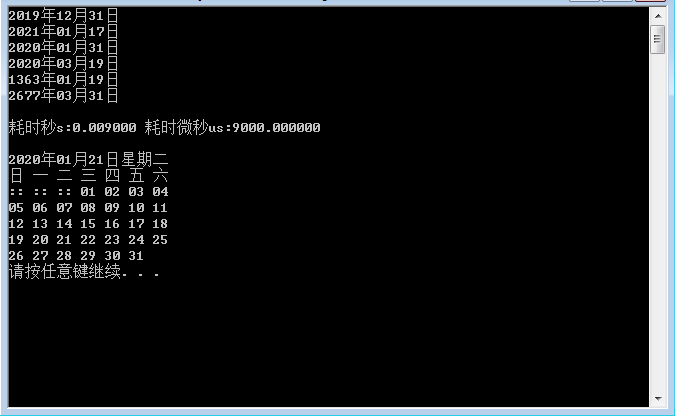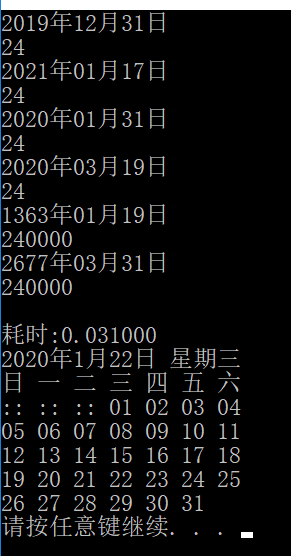### C++按月计算的天日期

```void 按月计算的天日期(int 年, int 月, int 日, int 天, char 运算符 = '-')

int 总天数 = 返回日期总天数(年, 月, 日);

if (运算符 == '-')while (日 <= 天)if (!--月)--年, 月 = 13; else 日 += 返回月的天数(年, 月);

//while (日 <= 天 || !月)if (!月)--年, 月 = 13; else 日 += 返回月的天数(年, --月);

if (运算符 == '+')while ((日 += 天) > 返回月的天数(年, 月 % 12) || 月 > 12)

if (月 > 12)++年, 月 = 1; else 日 -= 返回月的天数(年, 月++), 天 = 0;

日 -= 天;

cout << 年 << "年" << (月 < 10 ? "0" : "") << 月 << "月" << (日 < 10 ? "0" : "") << 日 << "日" << endl;

总天数 -= 返回日期总天数(年, 月, 日); cout << (总天数 < 0 ? -总天数 : 总天数) << endl;

}

按月计算的天日期(2020, 1, 24, 24);

按月计算的天日期(2020, 12, 24, 24, '+');

按月计算的天日期(2020, 2, 24, 24);

按月计算的天日期(2020, 2, 24, 24, '+');

按月计算的天日期(2020, 2, 24, 240000);

按月计算的天日期(2020, 2, 24, 240000, '+');
```